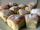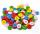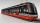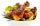# Pages of book

Lenka calculated that if every day to read 16 pages of the book, read a book a day earlier than had read only 14 pages a day. How many pages has this book?

Correct result:

n =  112

#### Solution:

16(d-1) = n
14 d = n

16•(d-1) = n
14•d = n

16d-n = 16
14d-n = 0

d = 8
n = 112

Our linear equations calculator calculates it.We would be pleased if you find an error in the word problem, spelling mistakes, or inaccuracies and send it to us. Thank you!Tips to related online calculators
Do you have a system of equations and looking for calculator system of linear equations?

## Next similar math problems:

• Hotel roomsIn the 45 rooms, there were 169 guests, some rooms were three-bedrooms and some five-bedrooms. How many rooms were?
• Waiting roomIn the waiting room are people and flies. Together they have 15 heads and 50 legs (fly has 6 legs). How many people and flies are in the waiting room?
• EquationsSolve following system of equations: 6(x+7)+4(y-5)=12 2(x+y)-3(-2x+4y)=-44
• The dormitoryThe dormitory accommodates 150 pupils in 42 rooms, some of which are triple and some are quadruple. Determine how many rooms are triple and how many quadruples.
• Linear systemSolve this linear system (two linear equations with two unknowns): x+y =36 19x+22y=720
• TreesAlong the road were planted 250 trees of two types. Cherry for 60 CZK apiece and apple 50 CZK apiece. The entire plantation cost 12,800 CZK. How many was cherries and apples?
• Two numbersWe have two numbers. Their sum is 140. One-fifth of the first number is equal to half the second number. Determine those unknown numbers.
• TheatroTheatrical performance was attended by 480 spectators. Women were in the audience 40 more than men and children 60 less than half of adult spectators. How many men, women and children attended a theater performance?
• Three friendsThe three friends spent 600 KC in a teahouse. Thomas paid twice as much as Paul. Paul a half less than Zdeněk. How many each paid?
• Equations - simpleSolve system of linear equations: x-2y=6 3x+2y=4
• Three brothersThe three brothers have a total of 42 years. Jan is five years younger than Peter and Peter is 2 years younger than Michael. How many years has each of them?
• DonutsIn the dining room at one table sit Thomas, Filip and Martin. Together they eat 36 donuts. How many donuts eaten everyone, when Filip ate twice as much as Thomas and Martin even half over Filip?
• ButtonsShirt has 6 buttons, blouse has 4 buttons. All buttons are 176. Shirts and blouses are together 36. How many are the shirts and blouses?
• Three daysDuring the three days sold in stationery 1490 workbooks. The first day sold about workbooks more than third day. The second day 190 workbooks sold less than third day. How many workbooks sold during each day?
• TramIn the three-part tram went 206 passengers in front of others, 226 after others and in the middle half of all. How many passengers went in tram total?
• Magic numberThe number 135 split to two addends so that one addend was 30 greater than 2/5 the addend.
• Farm2The farm bred turkeys, geese, hens and chickens. Everything is 400. Turkeys and geese a hen are 150. No hen has more than one chicken, but some hens has no chick. Half of them is also a quarter of the chickens. When the turkey subtracting 15, we get 75. H